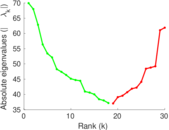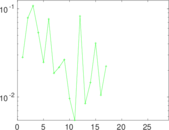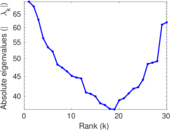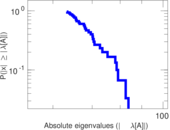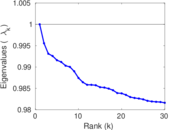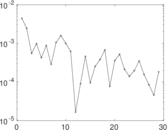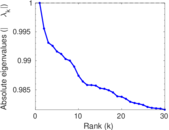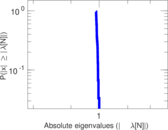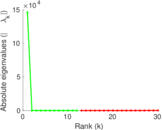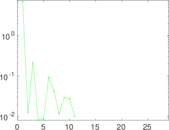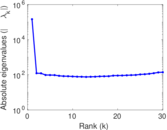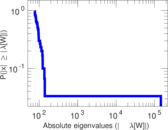# WordNet

This is the lexical network of words from the WordNet dataset. Nodes in the network are English words, and links are relationships between them, such as synonymy, antonymy, meronymy, etc. All relationships present in the WordNet dataset are included. The resulting network is undirected.

 Code `WO` Internal name `wordnet-words` Name WordNet Data source http://wordnet.princeton.edu/ AvailabilityDataset is available for download Consistency checkDataset passed all tests Category Lexical network Node meaning Word Edge meaning Relationship Network format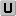Unipartite, undirected Edge typeUnweighted, no multiple edges LoopsDoes not contain loops

## Statistics

 Size n = 146,005 Volume m = 656,999 Loop count l = 0 Wedge count s = 35,863,116 Claw count z = 4,063,487,782 Cross count x = 624,320,669,873 Triangle count t = 1,144,910 Square count q = 32,926,054 4-Tour count T4 = 408,174,894 Maximum degree dmax = 1,008 Average degree d = 8.999 68 Fill p = 6.163 99 × 10−5 Size of LCC N = 145,145 Diameter δ = 16 50-Percentile effective diameter δ0.5 = 4.813 85 90-Percentile effective diameter δ0.9 = 6.318 86 Median distance δM = 5 Mean distance δm = 5.362 01 Gini coefficient G = 0.551 182 Balanced inequality ratio P = 0.295 863 Relative edge distribution entropy Her = 0.940 450 Power law exponent γ = 1.603 75 Tail power law exponent γt = 2.471 00 Tail power law exponent with p γ3 = 2.471 00 p-value p = 0.000 00 Degree assortativity ρ = −0.062 324 8 Degree assortativity p-value pρ = 0.000 00 Clustering coefficient c = 0.095 773 3 Spectral norm α = 69.934 4 Algebraic connectivity a = 0.022 464 9 Spectral separation |λ1[A] / λ2[A]| = 1.028 53 Non-bipartivity bA = 0.114 963 Normalized non-bipartivity bN = 0.046 198 2 Algebraic non-bipartivity χ = 0.079 502 3 Spectral bipartite frustration bK = 0.002 198 04 Controllability C = 15,096 Relative controllability Cr = 0.103 394

## Plots

### Fruchterman–Reingold graph drawing### Degree distribution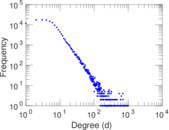### Cumulative degree distribution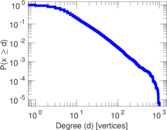### Lorenz curve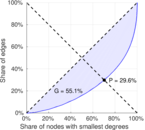### Spectral distribution of the adjacency matrix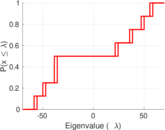### Spectral distribution of the normalized adjacency matrix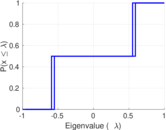### Spectral distribution of the Laplacian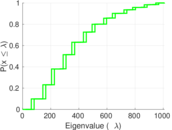### Spectral graph drawing based on the adjacency matrix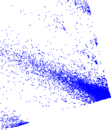### Spectral graph drawing based on the Laplacian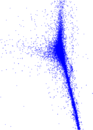### Spectral graph drawing based on the normalized adjacency matrix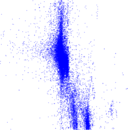### Degree assortativity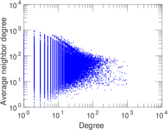### Zipf plot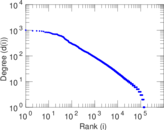### Hop distribution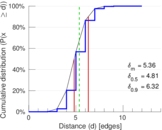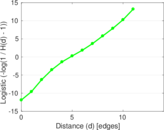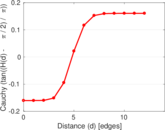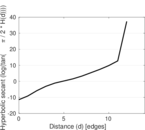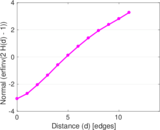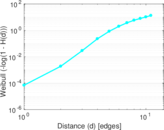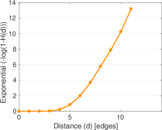### Clustering coefficient distribution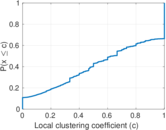### Average neighbor degree distribution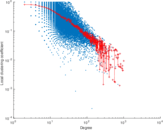### SynGraphy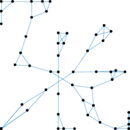### Matrix decompositions plots